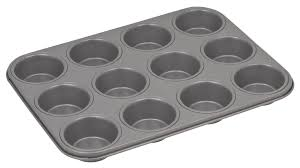# What Are Figurate Numbers? - Definition & Examples

Instructor: Elizabeth Hance

Elizabeth has taught elementary and middle school special education, and has a master's degree in reading education.

Do you know what a figurate number is? If you noticed the root word, 'figure', you are on the right track! In this lesson, you will learn about the different types of figurate numbers and how they form shapes.

## Understanding Figurate Numbers

A figurate number is a number that can be shown by taking points, or dots, and arranging them into a regular shape, such as a triangle or a pentagon. They are sometimes called polygonal numbers because they are arranged to form various polygons, or 2-dimensional shapes with three sides or more. For example, 10 is a figurate number. You can take ten points and arrange them to make a regular triangle.

Often, people think of figurate numbers as a series. You might see figurate numbers ordered to show how they grow. For example, take a look at the triangular numbers shown in this image: 1, 3, 6, 10, 15, and 21.## Triangular Numbers

Imagine a triangle with three dots. Each side is two dots long.

Now, imagine that you want to make a bigger triangle. Each side will be one dot longer. To make that triangle, you will need six dots.

How long would the next triangle's sides be? If you said, four dots, you are correct! To make a triangle with four dots for each side, your triangular number would be 10. To make even bigger triangles, keep adding a layer of dots to one side of your triangle so that it gets larger and larger, while all three sides remain the same length in dots.

## Rectangular & Square Numbers

Rectangular numbers are just like triangular numbers, except that they can be represented as rectangles. A rectangle has four sides, and the opposite sides are equal and parallel.

For example, 8, 12, and 15 are all rectangular numbers, but 5, 7, and 23 are not. If I gave you five round cupcakes, you could not arrange them into a rectangle. However, you could easily arrange 12 cupcakes in a rectangle.Square numbers are numbers that can be represented as…squares! They must have four equal sides. The smallest square number is 1. The largest square number is the product of any number multiplied by itself.

To unlock this lesson you must be a Study.com Member.

### Register to view this lesson

Are you a student or a teacher?

#### See for yourself why 30 million people use Study.com

##### Become a Study.com member and start learning now.
Back
What teachers are saying about Study.com

### Earning College Credit

Did you know… We have over 200 college courses that prepare you to earn credit by exam that is accepted by over 1,500 colleges and universities. You can test out of the first two years of college and save thousands off your degree. Anyone can earn credit-by-exam regardless of age or education level.